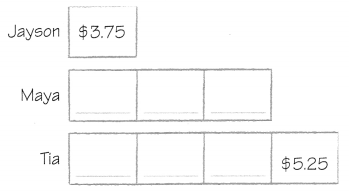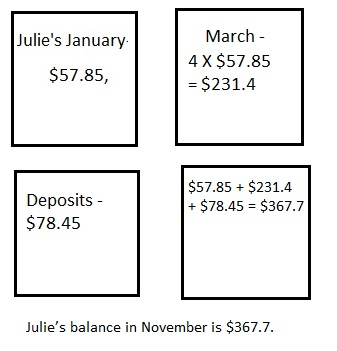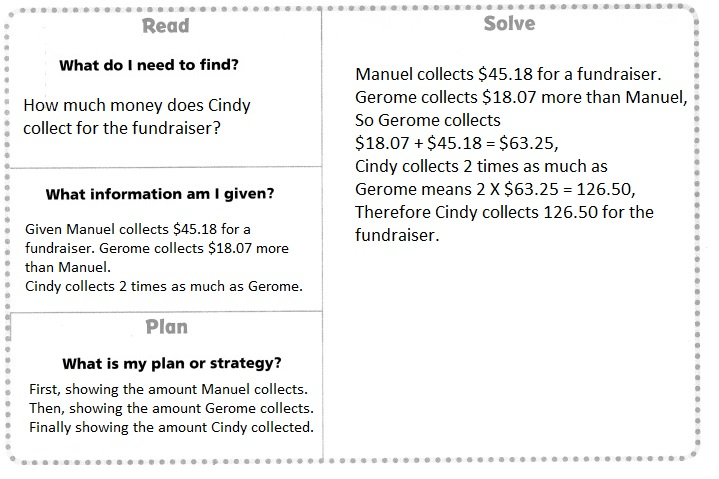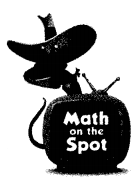# Texas Go Math Grade 5 Lesson 3.5 Answer Key Multiply Money

Refer to our Texas Go Math Grade 5 Answer Key Pdf to score good marks in the exams.
Test yourself by practicing the problems from Texas Go Math Grade 5 Lesson 3.5 Answer Key Multiply Money

## Texas Go Math Grade 5 Lesson 3.5 Answer Key Multiply Money

Essential Question
How can the strategy draw a diagram help you solve a decimal multiplication problem?

Unlock the Problem

A group of friends go to a local fair. Jayson spends S3.75.
Maya spends 3 times as much as Jayson. Tía spends $5.25 more than Maya. How much does Tia spend? Use the graphic organizer below to help you solve the problem. Read What do I need to find? I need to find ______Tia____ What information am I given? I need to use the amount spent by __Jayson_ to find the amount spent by and _Maya__ at the fair. plan What is my plan or strategy? I can draw a diagram to show ___Tia____ Solve The amount of money Maya and Tia spend depends on the amount Jayson spends. Draw a diagram to compare the amounts without calculating. Then, use the diagram to fìnd the amount each person spends.Jayson:$3.75
Maya: 3 × _$3.75__ = _$11.25__
Tia: _$11.25__ +$5.25 = __16.5__

So, Tia spent _$16.5_ at the fair. Try Another Problem Julie’s savings account has a balance of$57.85 in January.
By March, her balance is 4 times as much as her January balance.
Between March and November, Julie deposits a total of $78.45. If she does not withdraw any money from her account, what should Julie’s balance be in November?• How can the strategy draw a diagram help you solve a decimal multiplication problem? Answer: Julie’s balance in November$367.7,

Explanation:Math talk
Mathematical Processes

Describe a different diagram you could use to solve the problem.
Drawn diagram using Squares to solve the problem,

Explanation:Share and Show

Question 1.
Manuel collects $45.18 for a fundraiser. Gerome collects$18.07 more than Manuel.
Cindy collects 2 times as much as Gerome.
How much money does Cindy collect for the fundraiser?
First, draw a diagram to show the amount Manuel collects.
Then, draw a diagram to show the amount Gerome collects.
Next, draw a diagram to show the amount Cindy collects.
Finally, find the amount each person collects.
Cindy collects ___ for the fundraiser.Explanation:
Manuel collects $45.18 for a fundraiser. Gerome collects$18.07 more than Manuel,
So Gerome collects $18.07 +$45.18 = $63.25, Cindy collects 2 times as much as Gerome means 2 X$63.25 = $126.50, How much money does Cindy collect for the fundraiser? First, draw a diagram to show the amount Manuel collects. Then, draw a diagram to show the amount Gerome collects. Next, draw a diagram to show the amount Cindy collects. Finally, find the amount each person collects. Cindy collects$126.50 for the fundraiser.

Question 2.
What if Gerome collects $9.23 more than Manuel? If Cindy still collects 2 times as much as Gerome, how much money would Cindy collect? Answer: Cindy Collected$108.82,

Explanation:
Given Manuel collects $45.18 for a fundraiser, If Gerome collects$9.23 more than Manuel then
Gerome collected $45.18 +$9.23 = $54.41, Cindy collected 2 times as much as Gerome, So, 2 X$54.41 = $108.82, therefore money Cindy collected is$108.82.

Problem Solving

Question 3.
H.O.T. Multi-Step It costs $5.15 to rent a kayak for 1 hour at a local state park. The price per hour stays the same for up to 5 hours of rental. After 5 hours, the cost decreases to$3.75 per hour.
How much would it cost to rent a kayak for 6 hours?It costed $29.50 to rent a kayak for 6 hours, Explanation: Given It costed$5.15 to rent a kayak for 1 hour at a local state park.
The price per hour stays the same for up to 5 hours of rental.
After 5 hours, the cost decreases to $3.75 per hour. The cost to rent a kayak for 6 hours is for first 5 hours it is 5 X$5.15 = $25.15, for 6 hours it is$25.15 + $3.75 =$29.50.

Question 4.
H.O.T. Multi-Step Jenn buys a pair of jeans for $24.99. Her friend Karen spends$3.50 more for the same pair of jeans.
Vicki paid the same price as Karen for the jeans but bought 2 pairs.
How much did Vicki spend?
Vicki Spent $56.98 for 2 pair of jeans, Explanation: Given Jenn buys a pair of jeans for$24.99,
Her friend Karen spends $3.50 more for the same pair of jeans. Vicki paid the same price as Karen for the jeans but bought 2 pairs, So amount Vicki spent is 2($24.99 + $3.40) = 2($28.49) = $56.98. Daily Assessment Task Question 5. Apply A recipe for chili calls for three types of beans. How much will the beans cost if black beans are$12.15,
kidney beans are $16.27 and spicy refried beans are twice the cost of black beans? (A)$40.57
(B) $52.72 (C)$54.69
(D) $60.96 Answer: (B)$52.72,

Explanation:
Given a recipe for chili calls for three types of beans.
The beans cost if black beans are $12.15 + kidney beans are$16.27
+  spicy refried beans are twice the cost of black beans 2($12.15) =$12.15 + $16.27 +$24.30 = $52.72 matches with (B). Question 6. Use Math Language Ada and Chen are cooking different chili recipes. Ada spent$21.88 on ingredients while Chen spent twice as much as Ada.
What method could you use to find out how much Chen spent on ingredients?
(A) Divide $21.88 by 2. (B) Add$21.88, $14.44, and$14.44.
(C) Add $21.88,$21.88, and $21.88. (D) Multiply$21.88 by 2.
(D) Multiply $21.88 by 2, Explanation: Given using Math Language Ada and Chen are cooking different chili recipes, Ada spent$21.88 on ingredients while Chen spent twice as much as Ada.
Method we could use to find out how much Chen spent on ingredients is
Ada spent $21.88 and Chen has spent twice as much as Ada, So chen spent 2 X$21.88 which matches with (D) Multiply $21.88 by 2. Question 7. Multi-Step Marco spent$17.18 on tomatoes. Then he spent three times as
much on peppers. How much did he spend on tomatoes and peppers?
(A) $17.18 (B)$57.81
(C) $51.54 (D)$68.72
(D) $68.72, Explanation: Given Marco spent$17.18 on tomatoes. Then he spent three times as
much on peppers.
Marco spent on tomatoes and peppers is $17.18 + 3($17.18) =
$17.18 + 3 X$17.18,
$17.18 +$51.54 = $68.72 matches with (D). Texas Test Prep Question 8. At a bagel shop in town, each bagel costs$0.79.
If Mr. Thomas buys a box of 8 bagels,
how much will he pay for the bagels?
(A) $6.32 (B)$87.90
(C) $63.20 (D)$8.79
(A) $6.32, Explanation: Given at a bagel shop in town each bagel costs$0.79.
If Mr. Thomas buys a box of 8 bagels, The amount he will pay
for the bagels is $0.79 X 8 =$6.32, Matches with (A).

### Texas Go Math Grade 5 Lesson 3.5 Homework and Practice Answer Key

Question 1.
Brandy buys 6 packs of party favors. The favors cost $3.49 per pack. She pays$1.26 in sales tax. How much does Brandy spend on the party favors?
Brandy spent on the party favors $22.2, Explanation: Given Brandy buys 6 packs of party favors. The favors cost$3.49 per pack.
She pays $1.26 in sales tax. Therefore Brandy spent on the party favors is 6 X$3.49 + $1.26 =$20.94 + $1.26 =$22.2.

Question 2.
Hector sells his used books at a garage sale for $2.75 each. On Saturday, he sells 3 books. On Sunday, he sells 7 books. How much does Hector make selling his books? Answer: Hector made$27.5 by selling his books,

Explanation:
Given Hector sells his used books  at a garage sale for $2.75 each, On Saturday he sells 3 books- So collected 3 X$2.75 = $8.25, On Sunday, he sells 7 books- So collected 7 X$2.75 = $19.25, Hector made$8.25 + $19.25 =$27.5 by selling his books.

Question 3.
The original price for a case of paper is $12.88. The case of printer paper is on sale for 0.5 times the original price. How much will Alison spend on 5 cases of printer paper if she buys them on sale? Answer: Alison spent on 5 cases of printer paper if she buys them on sale is$32.2,

Explanation:
Given the original price for a case of paper is $12.88. The case of printer paper is on sale for 0.5 times the original price. The price of case of printer paper is$12.88 X 0.5 = $6.44, As Alison spend on 5 cases of printer paper if she buys them on sale is 5 X$6.44 = $32.2. Question 4. Jordan has$14.56 in her wallet. Nico has three times as much
money as Jordan in his wallet.
Avery has $5.25 more than Nico. How much money does Avery have? Answer: Avery have$48.93 in all,

Explanation:
Given Jordan has $14.56 in her wallet. Nico has three times as much money as Jordan in his wallet, So Nico has 3 X$14.56 = $43.68, As Avery has$5.25 more than Nico, So money does Avery have
is $43.68 +$5.25 = $48.93. Question 5. In September, Ellen deposits$52.00 in her savings account. The next month,
she deposits $22.50 more in her account. In November, Ellen deposits four times the amount she deposits in October. How much does Ellen deposit in her account in the three months? Answer: Ellen deposited in her account in three months is$424.5,

Explanation:
Given in  September Ellen deposits $52.00 in her savings account, The next month she deposited$22.50 more in her account,
In October she had $52.00 +$22.50 = $74.50, As in November Ellen deposited four times the amount she deposited in October, So in November, Ellen deposited 4 X$74.50 = $298, In total Ellen deposited in her account in three months is$52.00 + $74.50 +$298 = $424.5. Question 6. Leticia buys a magazine and rents 2 movies for her weekend entertainment. The price of a movie rental is$4.95. The magazine costs $5.99. How much does Leticia spend on her weekend entertainment? Answer: Leticia spent on her weekend entertainment is$14.85,

Explanation:
Given Leticia buys a magazine and rents 2 movies for her weekend entertainment.
The price of a movie rental is $4.95. The magazine costs$5.99.
Rents 2 movies for her weekend entertainment is 2 X $4.95 =$9.9
Leticia spent on her weekend entertainment is $4.95 +$9.9 = $14.85. Problem Solving Question 7. Sam and Justin each rent a bike for 4 hours. The rental price for 1 bike is$4.35 per hour.
Then the boys decide to ride together on a tandem bike for another hour for $6.75. What is the total cost of the boys’ bike rental? Answer: The total cost of the boy’s bike rental is$41.55,

Explanation:
Given Sam and Justin each rent a bike for 4 hours,The rental price for 1 bike is $4.35 per hour. Sam rented price is 4 X$4.35 = $17.4, Similarly Justin rented price is$17.4,
As the boys decided to ride together on a tandem bike for another hour for $6.75 the total cost of the boy’s bike rental is$17.4 + $17.4 +$6.75 = $41.55. Question 8. Kevan spends$19.99 at the mall. Amir spends $2.99 more than Kevan. Raul spends twice as much as Amir. How much does Raul spend? Answer: Raul spends$45.96,

Explanation:
Given Kevan spends $19.99 at the mall. Amir spends$2.99 more than Kevan.
So Amir spended $19.99 +$2.99 = $22.98 Raul spends twice as much as Amir. Therefore Raul spends 2 X$22.98 = $45.96. Texas Test Prep Fill in the bubble completely to show your answer. Question 9. Amelia spent$3.16 on peaches. Then she spent four times as much on raspberries.
How much did she spend on peaches and raspberries?
(A) $12.64 (B)$15.80
(C) $6.32 (D)$8.50
(B) $15.80, Explanation: Given Amelia spent$3.16 on peaches. Then she spent four times as much on raspberries,
So raspberries she has spent is 4 X $3.16 =$ 12.64, Now Amelia spent on
peaches and raspberries is $3.16 +$12.64 = $15.80 which matches with (B). Question 10. Ryan buys 3 cans of tennis balls for$2.67 each. He buys 2 pairs of socks for $3.55 each. He pays with a$20 bill. How much change does Ryan get?
(A) $2.64 (B)$15.11
(C) $13.78 (D)$4.89
(D) $4.89, Explanation: Given Ryan buys 3 cans of tennis balls for$2.67 each, So Ryan buys for
3 X $2.67 =$8.01, Ryan buys 2 pairs of socks for $3.55 each it costed 2 X$3.55 = $7.10, He pays$20 and he gets a change of $20 – ($8.01+ $7.10), =$20 – $15.11 =$4.89 matches with (D).

Question 11.
Sonya counts the coins in her coin purse. She has 7 quarters, 11 dimes, and 15 nickels.
She has two more pennies than the number of quarters and dimes combined.
What is the value of Sonya’s coins?
(A) $3.80 (B)$3.65
(C) $3.70 (D)$3.95
(A) $3.80, Explanation: Given Sonya counts the coins in her coin purse. She has 7 quarters, 11 dimes and 15 nickels. 7 quarters X 1/40 =$0.175,
11 dimes X 0.01 = $0.11, 15 nickels X 0.05 =$0.75,
= $0.175 +$0.11 + $0.75 =$1.035,
She has two more pennies than the number of quarters and dimes combined is
(2 X $0.01) +$0.175 + $0.11 =$0.02 + $0.175 +$0.11 = 0.305,
The value of Sonya’s coins is (A) $3.80. Question 12. Chef Michaels spends$24.46 on ingredients for a cake and $13.92 on ingredients for a pie. What method could you use to find out how much Chef Michaels spends on ingredients for the cake if he doubles the recipe? (A) Add$24.46 and $13.92. (B) Add$24.46, $24.46, and$13.92.
(C) Multiply $24.46 by 2. (D) Divide$24.46 by 2.
(B) Add $24.46,$24.46, and $13.92, Explanation: Given Chef Michaels spends$24.46 on ingredients for a cake and
$13.92 on ingredients for a pie. The method we could use to find out how much Chef Michaels spends on ingredients for the cake if he doubles the recipe is adding$24.46 and $24.46 and$13.92 which matches with (B).

Question 13.
Multi-Step At the farmer’s market, heirloom tomatoes cost $5.99 a pounds beefsteak tomatoes cost$3.19 a pound, and cherry tomatoes cost $2.99 a pound. Joe bought 2 pounds of each type of tomato. How much did he spend on tomatoes? (A)$21.35
(B) $12.17 (C)$24.34
(D) $18.16 Answer: (C)$24.34,

Explanation:
Given at the farmer’s market heirloom tomatoes cost $5.99 pounds, beefsteak tomatoes cost$3.19 a pound and cherry tomatoes cost $2.99 a pound. Joe bought 2 pounds of each type of tomato, heirloom tomatoes cost 2 X$5.99 pound = $11.98, heirloom tomatoes cost 2 X$5.99 pound = $6.38, cherry tomatoes cost 2 X$2.99 a pound = $5.98, Total tomatoes cost are$11.98 + $6.38 +$5.98 = $24.34. Question 14. Multi-Step Carnations cost$0.99 each, daisies cost $1.75 each, and lilies cost$2.26 each.
How much would an arrangement cost that is made with 3 carnations,
two more daisies than carnations, and twice as many lilies as daisies?
(A) $34.32 (B)$15.51
(C) $25.28 (D)$40.59
(D) $40.59, Explanation: Given Carnations cost$0.99 each, daisies cost $1.75 each and lilies cost$2.26 each.
The arrangement cost that is made with 3 carnations is 3 X ($0.99 +$1.75 + $2.26) = = 3 X$5.00 =  $15.00, Now two more daisies than carnations is (2 X$1.75) + $15.00 =$3.50 + $15.00 =$18.50 and
twice as many lilies as daisies is (2 X $2.26) +$1.75 = $4.52 +$1.75 = $6.27, therefore arrangement cost is$15.00 + $18.50 +$6.27 =\$40.59.